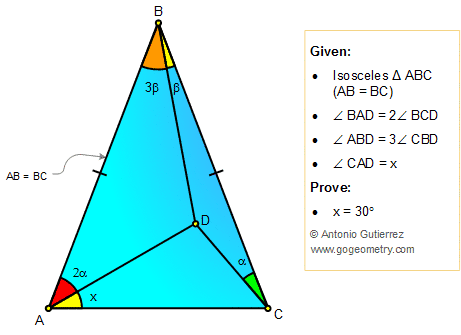# Geometry Problem 45 with Solution: Angles, Triangle, Double Angle, Triple Angle, 30 Degrees

< PREVIOUS PROBLEM  |  NEXT PROBLEM >

In the figure,  AB = BC, the measure of angle BAD is double the measure of angle BCD and the measure of angle ABD is triple the measure of angle CBD. Prove that x = 30°.Home | Geometry | Problems | Open Problems | 10 Problems | 41-50 | Double Angle | Email | By Antonio Gutierrez Post or view a solution to the problem 45